• 本文主要讲述以Qt做主语言，MATLAB做数学计算，并将计算的结果生成相应的库文件或者直接生成一个可执行文件给Qt调用，以此来实现QtMATLAB混合编程，资源包含详细的解释代码以供参考。
• Qt 5.9与MATLAB 2017b混合编程的简单实例，包括Qt 5.9项目的全部源程序
• Qtmatlab混合编程.m文件示例Qt工程中引用matlab相关的库文件Qt中调用matlab的几种方法1.动态库方法maltlab函数编译动态库Qt中调用matlab生成的动态库小结2. Qt调用matlab引擎Qt启动matlab引擎Qt和matlab之间数据...


Qt与matlab混合编程
.m文件示例Qt工程中引用matlab相关的库和文件Qt中调用matlab的几种方法1.动态库方法maltlab函数编译动态库Qt中调用matlab生成的动态库小结
2. Qt调用matlab引擎Qt启动matlab引擎Qt和matlab之间数据交互关闭matlab引擎其他matlab函数中有多个返回值时Qt指令写法直接调用.m文件
mxArray类型与C++数组互转二维数组转mxArray*mxArray*转二维数组
小结
3.使用txt等中间文件在Qt和matlab之间进行数据交互

以下内容使用的操作系统、软件及编译器版本：win10、Qt5.9.6(msvc2015_64)、matlab R2018b

.m文件示例
function [C]= matAdd(A, B)
% 两个矩阵数相加
C = A + B;
end

Qt工程中引用matlab相关的库和文件
.pro文件作如下设置，其中 D:/MATLAB/R2018b 为matlab的安装路径，可以根据自身实际安装的路径进行更改
# .h文件
INCLUDEPATH += D:/MATLAB/R2018b/extern/include
INCLUDEPATH += D:/MATLAB/R2018b/extern/include/Win64

# MATLAB 的.lib库文件
INCLUDEPATH += D:/MATLAB/R2018b/extern/lib/win64/microsoft
DEPENDPATH += D:/MATLAB/R2018b/extern/lib/win64/microsoft

win32: LIBS += -LD:/MATLAB/R2018b/extern/lib/win64/microsoft/-llibmex
win32: LIBS += -LD:/MATLAB/R2018b/extern/lib/win64/microsoft/-llibmx
win32: LIBS += -LD:/MATLAB/R2018b/extern/lib/win64/microsoft/-llibmat
win32: LIBS += -LD:/MATLAB/R2018b/extern/lib/win64/microsoft/-llibeng
win32: LIBS += -LD:/MATLAB/R2018b/extern/lib/win64/microsoft/-lmclmcr
win32: LIBS += -LD:/MATLAB/R2018b/extern/lib/win64/microsoft/-lmclmcrrt

Qt中调用matlab的几种方法
1.动态库方法
将.m文件编译成C++可以直接调用的动态库，之后在Qt程序中直接使用。这种方法最为简单，也最为常见。
maltlab函数编译动态库
matlab中打开需要编译的.m文件，命令行中输入
mbuild -setup输入命令后回车，根据弹出的选项进行对应的选择之后在命令行中输入
deploytool

在弹出的窗口中选择 Library Compiler之后在弹出的窗口中选择要编译的动态库的类型，点击 + 添加.m文件（可一次添加多个），设置生成的动态库的名称，保存当前工程（若此时不保存，在点击生成之前系统会提示保存），最后点击 Package 生成动态库。生成成功后，在matlab工程下将看到生成的动态库Qt中调用matlab生成的动态库
Qt工程中.pro引用生成的动态库和对应头文件
LIBS += F:/Programs/QT/MatlabTest/link/matAdd.lib

Qt程序中调用动态库中的函数
if (!matAddInitialize()) // DLL初始化
{
qDebug() << "DLL initialization failed";
return;
}

double vectA[]={1,2,3};  // 向量A
double vectB[]={5,6,7};  // 向量B

int rowCnt=1;   // 行数
int colCnt=3;   // 列数
int elementCnt=3;  // 元素个数

mwArray matrixA(rowCnt, colCnt, mxDOUBLE_CLASS, mxREAL);  // 定义数组
matrixA.SetData(vectA, elementCnt);  // 将C++ 的一维数组存储到MATLAB的二维数组

mwArray matrixB(rowCnt, colCnt, mxDOUBLE_CLASS, mxREAL);
matrixB.SetData(vectB, elementCnt);

// 计算
mwArray matrixC(rowCnt, colCnt, mxDOUBLE_CLASS, mxREAL);  // 定义数组，double类型
int nargout=1;  // 输出变量个数
matAdd(nargout, matrixC, matrixA, matrixB);  // 调用函数计算，返回的结果保存在matrixC变量中

// 读取结果
int dim = 1;  // 按照向量读出matrixC
double av = matrixC.Get(dim, 1);  // 第1个元素
double bv = matrixC.Get(dim, 2);  // 第2个元素
double cv = matrixC.Get(dim, 3);  // 第3个元素

//    int dim = 2; //按照二维数组读出matrixC
//    double av = matrixC.Get(dim, 1, 1); //第1行，第1列
//    double bv = matrixC.Get(dim, 1, 2); // 第1行，第2列
//    double cv = matrixC.Get(dim, 1, 3); // 第1行，第3列


小结
在Qt中使用matlab编译生成的动态库是最为简单和常见的方法，但是在一些情形下matlab无法将程序编译成动态库（脚本程序或某些函数不支持编译等），这种情况下则必须采用其它办法在C++中调用matlab程序。
2. Qt调用matlab引擎
在Qt中可以直接启动matlab引擎，此后可从Qt程序中向matlab发送指令进行相关操作，指令的语法和matlab命令窗口语法一致。
Qt启动matlab引擎
头文件需要包含
#include "engine.h"

启动matlab引擎
Engine *ep = nullptr;
if(!(ep = engOpen(nullptr)))  // 启动matlab引擎
{
qDebug() << "无法启动matlab引擎";
return;
}
engSetVisible(ep, false);  // 隐藏matlab自带命令窗口

其中，当matlab引擎启动的时候，默认是会弹出matlab的命令行窗口的，此时若不需要，可以使用 engSetVisible(ep, false) 函数将该窗口隐藏。Qt和matlab之间数据交互
// 给变量赋值
double init1 = 3.0;
mxArray *a = nullptr;
a = mxCreateDoubleMatrix(1, 1, mxREAL);
memcpy((void*) mxGetPr(a), (void*)&init1, sizeof (double));
engPutVariable(ep, "A", a);
mxDestroyArray(a);

double init2 = 1.0;
mxArray *b = nullptr;
b = mxCreateDoubleMatrix(1, 1, mxREAL);
memcpy((void*) mxGetPr(b), (void*)&init2, sizeof (double));
engPutVariable(ep, "B", b);
mxDestroyArray(b);

engEvalString(ep, "cd F:/Programs/others/MatlabTest");  // 向matlab发送字符串命令

mxArray *result = engGetVariable(ep, "one");   //将MATLAB结果传回qt

qDebug() << *mxGetPr(result);  // 打印结果

engEvalString 函数用于从Qt向matlab发送命令，engGetVariable 函数用于获取matlab计算得到的结果，返回值是 mxArray* 类型，mxGetPr 用于将matlab支持的mxArray类型转换为C++支持的数据类型（double*)。
关闭matlab引擎
engClose(ep);  // 关闭matlab引擎

其他
matlab函数中有多个返回值时Qt指令写法
.m文件
function [C, D]= matAdd(A, B)
% 两矩阵相加和相减
C = A + B;
D = A - B;
end

Qt程序写法如下，指令的语法和在matlab命令行中写法一致。
engEvalString(ep, "[one, two]=matAdd(A,B);");
mxArray *result = engGetVariable(ep, "one");   //将MATLAB结果传回qt
mxArray *result1 = engGetVariable(ep, "two");

直接调用.m文件
当.m文件中是脚本程序时，可以直接执行.m文件
engEvalString(ep, "load('XXX/XXX/XXX/xxx.m');");

mxArray类型与C++数组互转
上面的示例中传入matlab的变量只是两个double型的数（也可以理解为1行1列的矩阵），当传入或者返回的数据是多行多列的矩阵时，写法与上文略有不同。
二维数组转mxArray*
   double init3 = {{1, 2, 3, 4, 5},{11, 22, 33, 44, 55}};
mxArray *c = nullptr;
c = mxCreateDoubleMatrix(2, 5, mxREAL);
memcpy((void*) mxGetPr(c), (void*)init3, sizeof (init3));

mxArray*转二维数组
int M = mxGetM(c);  // 行数
int N = mxGetN(c);  // 列数
double *ans = mxGetPr(c);  // 转为数组类型

// 打印数组结果
for(int i = 0; i < M; ++i)
{
for(int j = 0; j < N; ++j)
{
cout << *(ans + (i * N + j)) << " ";
cout.flush();
}
cout << "\n";
}

小结
使用Qt调用matlab引擎方法比较比较灵活，相当于在Qt中打开了一个matlab，但是matlab引擎启动较慢，并且需要进行二者之间的数据交互，容易出错，此外还需要一定的matlab语法基础。
3.使用txt等中间文件在Qt和matlab之间进行数据交互
这种方法比较简单，Qt和matlab两者基本互相独立，将Qt获取到的数据保存到txt等中间文件当中，matlab程序中需要的参数从txt文件中读取，并将返回结果同样保存为txt文件，供Qt程序读取和显示。 （若在运行Qt程序过程中，全程不想打开matlab软件，则可以使用Qt启动带命令窗口的matlab引擎，之后的操作在命令行窗口中进行。）
简单粗暴最有效。
展开全文c++
• 文档详细的介绍了QTMatlab混合编程，网上资料很少，所以整理了一下给刚入门的朋友学习之用
• test2中是MATLAB写的函数f.m CC++文件夹分别是生成的C或C++的动态链接库等文件 CTest2是Qt的工程 需要修改的地方有很多，首先是.pro文件中的路径， 然后运行有错误的地方可以先备注掉， 将dllctf文件复制到运行...
• 行业-电子政务-基于Qt和MATLAB混合编程的冷热电联供运行监控与能量管理系统研发.zip
• 师兄留下了一些matlab编写的程序，老师希望用Qt绘制界面，matlab完善算法功能，两者混合编程。任务布置下来，对于编程能力薄弱的我是一个极大的挑战，参考网上很多大佬的文档，初步实现了一个小小的自测试混合编程，...
1. 内容简介
师兄留下了一些matlab编写的程序，老师希望用Qt绘制界面，matlab完善算法功能，两者混合编程。任务布置下来，对于编程能力薄弱的我是一个极大的挑战，参考网上很多大佬的文档，初步实现了一个小小的自测试混合编程，中间也遇到了很多问题，在此记录一下，给自己警醒也希望可以给后来者提供一些帮助。
2. 软件环境
2.1QT编译器
软件环境很重要，不然很容易遇到各种问题，很多大佬实现混合编程时在QT中使用的是MSVC编译器，考虑到我这边有一部分Qt程序已经使用MinGW编写好了，不想再切换成MSVC编译修改，所以决定使用Qt5.10.1的MinGW编译器（32位）。
2.2Matlab2015b（32位）
2.3Visual studio 2015
（附：Matlab2015b破解版和Vs2015安装包可在我的资源中搜索，两份安装包放在一起了，为永久免费百度网盘链接，存储在txt中上传的，有需要的朋友可以自行下载，QT的安装包可自行去QT官网下载）
3.主要流程
3.1如何在Matlab中将m文件编译为C++语言的DLL文件
3.2如何在Qt项目中加入自定义DLL相关的LIB文件，以及MATLAB相关的LIB文件和H文件搜索路径
3.3MATLAB运行时DLL文件所在路径，及系统相关的环境变量设置
3.4如何在Qt中调用自定义DLL中的函数，如何通过mwArray类传递输入输出参数
4.具体步骤
4.1Matlab中m文件编译为C++语言的DLL文件
在MATLAB中编写一个简单的myFunc.m，其代码如下：

function C=myFunc(A,B) C=A+B; end

在编译m文件之前，需要保证MATLABCompiler已经设置好了编译器，在MATLAB命令行窗口中使用mbuild –setup 设置使用C编译器。

mbuild -setup

MBUILD 配置为使用 ‘Microsoft Visual C++ 2015 ©’ 以进行 C 语言编译。 要选择不同的语言，请从以下选项中选择一种命令:

mex -setup C++ -client MBUILD mex -setup FORTRAN -client MBUILD

要使用C++编译器，就用命令 mbuild –setup C++ 设置

mbuild -setup C++ MBUILD 配置为使用 ‘Microsoft Visual C++ 2015’ 以进行 C++ 语言编译。

我的电脑上安装了Visual Studio 2015，MATLAB会自己查找可用的编译器，若没有自动找到编译器，可能版本兼容问题或其他问题，可搜索相关资料解决问题。 在命令行中输入 deploytool，出现如下的对话框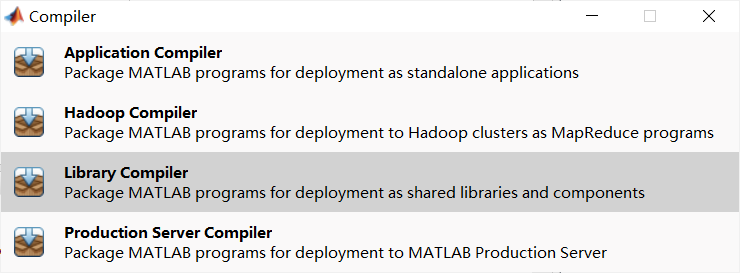“ApplicationCompiler”用于将m文件编译为exe文件直接运行，“Library Compiler”用于将m文件编译为DLL、COM组件等形式。我们要生成DLL文件，所以选择“Library Compiler”。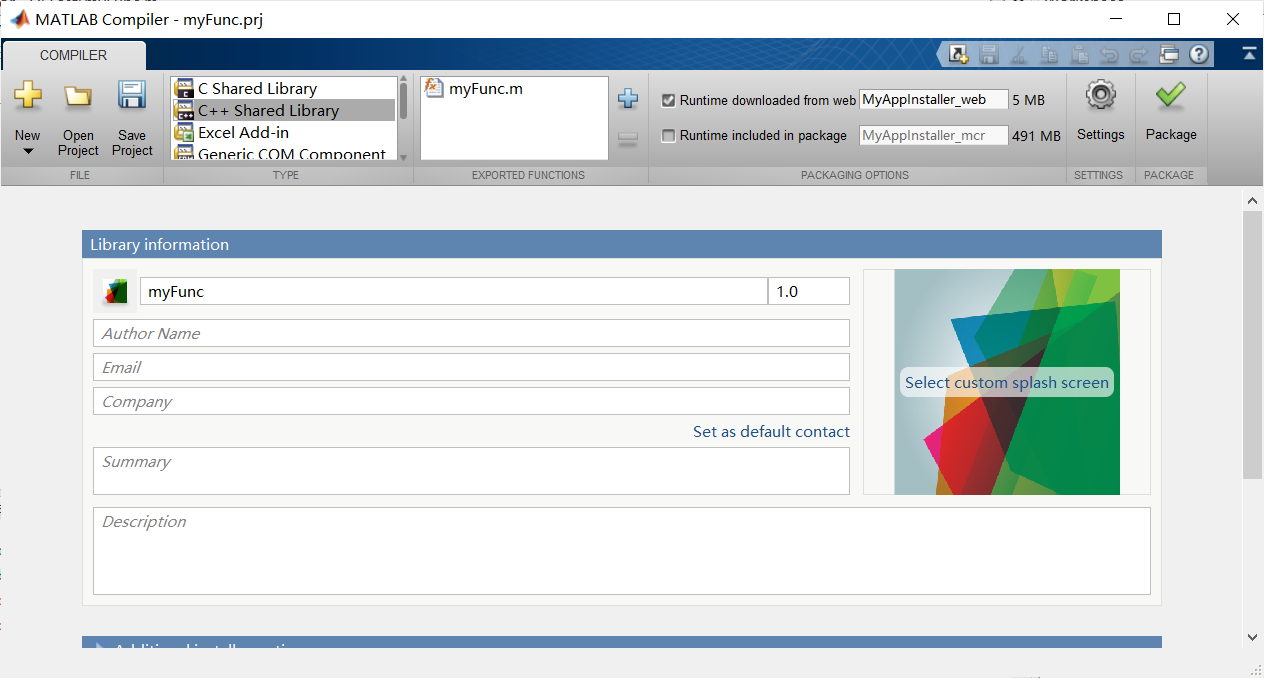在上图的MATLAB Compiler窗口中，“TYPE”部分选择C++ shared Library，“EXPORTED FUNCTIONS”是需要导出的m文件，点击右侧的“Add”按钮选择编写的文件myFunc.m。右侧是MATLAB运行时库的安装打包方式，在本机上测试可选择“Runtime downloaded from web”。 保存项目为myFunc.prj，然后点击“Package”按钮进行编译和打包。 打包完成后，在项目文件myFunc.prj的目录下生成与项目同名的子目录，即\myFunc，该目录下有3个文件夹。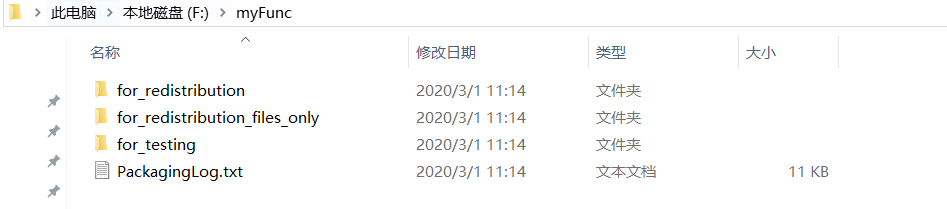muFunc\for_redistribution_files_only目录下是编译生成的.dll 、.lib和.h文件，其中.lib和.h文件是在Qt项目编译时需要用到的，.dll文件是程序运行时需要用到的。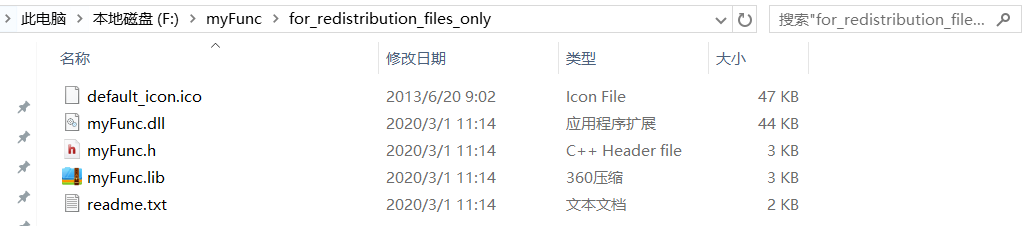4.2 Qt 5.10.1项目中使用myFunc.dll
4.2.1Qt项目创建
创建一个QtWidget Application项目test01，类名是Test，主窗口基于QWidget。
4.2.2库文件myFunc.lib 的加入
在项目目录下新建一个include目录，将前面编译生成的myFunc\for_redistribution_files_only目录下的myFunc.lib，myFunc.h文件复制到此目录下。 在Qt Creator里，myFunc项目节点上单击右键，选择“Add Library…”，在出现的向导中首先选择“External Library”，后续出现下图的界面，选择库myFunc\include\目录下的库文件matLib，其他选择如图。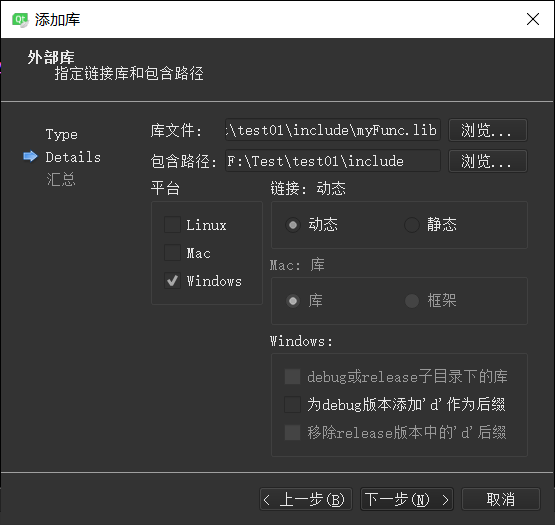在此对话框完成后，在test01.pro文件中会增加如下的几行：
win32: LIBS += -L$$PWD/include/ -lmyFunc INCLUDEPATH +=$$PWD/include
DEPENDPATH += $$PWD/include  4.2.3Matlab其他依赖库和头文件搜索路径的加入 除了自己编译生成的DLL相关的.lib文件和头文件，要编译此Qt项目，还需要用到MATLAB的几个.lib文件和.h文件。 我的电脑上，MATLAB2015b安装在C:\Program Files (x86)\MATLAB\R2015b目录下，在test01.pro文件中需要加入如下的设置： INCLUDEPATH +=$$quote(C:/Program Files (x86)/MATLAB/R2015b/extern/include)

DEPENDPATH += $$quote(C:/Program Files (x86)/MATLAB/R2015b/extern/include) LIBS+= -L$$quote(C:/Program Files (x86)/MATLAB/R2015b/extern/lib/win32/microsoft) -llibmx

LIBS+= -L$$quote(C:/Program Files (x86)/MATLAB/R2015b/extern/lib/win32/microsoft) -llibmat LIBS+= -L$$quote(C:/Program Files (x86)/MATLAB/R2015b/extern/lib/win32/microsoft) -lmclmcr

LIBS+= -L$$quote(C:/Program Files (x86)/MATLAB/R2015b/extern/lib/win32/microsoft) -lmclmcrrt  除此之外，由于使用的时MinGW的编译器，还需要在头文件中添加 DEFINES += __MW_STDINT_H__  这个东西是针对matlab的mclmcr.h头文件中第170行到第190行中编译器的一些宏的设定，一般来说如果是mingW编译器我们一般写+= MING_W，但是这个头文件中并没有给出mingW编译器的情况，所以一般选择加上上面这行代码，选择一个编译器的类型。 4.2.4系统环境变量的设置 额外加入的一些库实际上对应于MATLAB运行时的一些DLL文件，这些运行时文件主要在以下几个目录下，所以要保证这些目录添加到了Windows的环境变量PATH里。 C:\Program Files (x86)\MATLAB\R2015b\runtime\win32; C:\Program Files (x86)\MATLAB\R2015b\bin C:\Program Files (x86)\MATLAB\R2015b\extern\lib\win32\microsoft D:\Qt\5.10.1\msvc2015\bin 4.2.5编写使用DLL内函数myFunc()的代码  QLibrary myLib("myFunc.dll"); typedef bool MW_CALL_CONV(*Fun)(int,class mwArray const &,class mwArray const &,class mwArray const &); Fun myFunc = (Fun) myLib.resolve("?myFunc@@YAXHAAVmwArray@@ABV1@1@Z"); if(!myFuncInitialize()) { qDebug()<<"could not initialize libmyfuncdll\n"; exit(0); } QString result; double para1,para2; para1=ui->lineEdit->text().toDouble(); para2=ui->lineEdit_2->text().toDouble(); mwArray a(1,1,mxDOUBLE_CLASS); mwArray b(1,1,mxDOUBLE_CLASS); mwArray c(1,1,mxDOUBLE_CLASS); a(1,1)=para1; b(1,1)=para2; myFunc(1,c,a,b); result=c.ToString(); ui->lineEdit_3->setText(result);  还要记得在test.h头文件中添加 #include <QLibrary> #include "myFunc.h" #include <QDebug>  最后，将myFunc.dll文件复制粘贴到编译生成的debug文件夹下，到此为止，所有准备工作都完成了。 对于 QLibrary myLib("myFunc.dll"); typedef bool MW_CALL_CONV(*Fun)(int,class mwArray const &,class mwArray const &); Fun myFunc = (Fun) myLib.resolve("?myFunc@@YAXHAAVmwArray@@ABV1@1@Z");  需要说明的是由于matlab使用vs的msvc编译器生成的dll文件，生成后我们使用mingW调用，但生成的头文件中指定各种编译器对应的情况，但唯独没有MinGW，这就导致了在使用MinGW编译器时我们使用了： DEFINES += MW_STDINT_H 使用msvc编译器调用matlab生成的dll时，编译器会自动识别函数名，因此也不需要使用resolve函数。 4.2.6编译和运行 在lineEdit中输入任意数字，点击计算按钮，就可以看到结果了！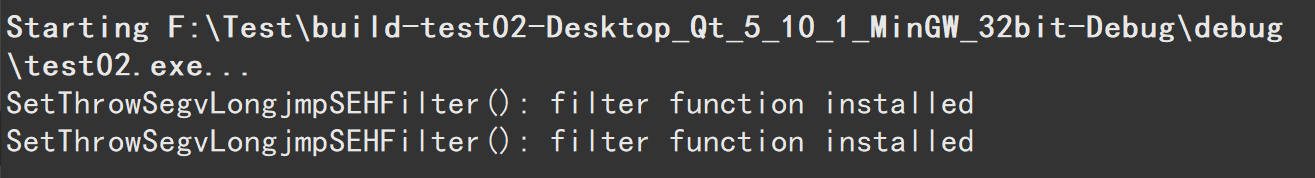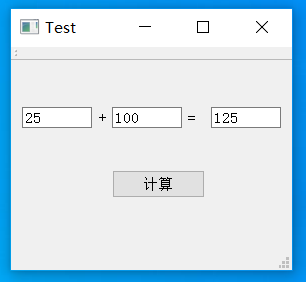5 特别提醒 刚开始向别人学习按照步骤操作的时候，遇到了一些问题和注意事项： 1、Qt的编译器一定要注意，是MSVC还是MinGW很重要，不同的编译器需要添加的代码是不一样的，如果Qt选择MSVC64位的，电脑安装的VS也要是64位的，并且Matlab的版本年份一般要高于VS的版本年份，比如选择matlab2017b和VS2015两个64位进行搭配，避免在mbuild-setup时出现matlab无法识别VS的情况。 2、编译没有错误，运行的时候出现强制结束程序。可能是如下两个原因： 第一个原因：请参考https://blog.csdn.net/hongandyi/article/details/79427078 第二个原因：请下载一个的Dependency Walker 2.2查看dll文件，resolve的文件名是否一致，程序强制退出，这个问题卡了我好久，后来发现下面的@1@Z，我打成了@@Z。改正过来，程序正常运行。 QLibrary myLib(“myFunc.dll”); typedef bool MW_CALL_CONV(*Fun)(int,class mwArray const &,class mwArray const &,class mwArray const &); Fun myFunc = (Fun) myLib.resolve("?myFunc@@YAXHAAVmwArray@@ABV1@1@Z"); 建议参考：https://blog.csdn.net/Justice132/article/details/83830151 6 代码链接 https://download.csdn.net/download/China_Rocky/12205984 7 总结 Qt调用matlab动态链接库 好处： 1、qt做界面，matlab做算法，二者相得益彰 2、相对于将算法C++化，这样做当然节约时间和成本 缺点： 程序执行速度慢，容易出错，步骤比较繁琐 补充：后来遇到一个问题，就是matlab中有多个m文件，m1文件是主程序文件，它的函数参数是其他m文件的函数，而不是矩阵或数组，在调用过程中编译没有出错，运行时程序崩溃。如果遇到这种情况，是因为参数类型不匹配的关系，声明中的参数是矩阵类型，而代入的实参是函数，只需要把matlab程序中的函数参数去掉，重新生成dll文件并替换掉include文件夹中原来的dll文件，然后在Qt中直接调用即可。 谢谢链接中诸多大佬的总结，通过学习，避免了很多问题，但是自己在实现的过程中，也遇到了各种各样的问题，看到网上主要是QtMSVC编译下matlab混合编程比较多，而MinGW编译下的混合编程较少，步骤流程有欠完善，所以写了这篇文章，也是我写的第一篇博客，希望可以帮助到有需要的朋友！不足之处，还请多多指教！ 展开全文经验分享 编译器 c++ • 演示Qt 5.9与Matlab 2017b混合编程中，用于传递数据的mwArray数组的使用方法，包括数组维数设置、传入数据、读取返回数据、字符串型数据等。博文地址 https://blog.csdn.net/HongAndYi/article/details/79477031 • ## Qt与matlab混合编程详细实现过程 千次阅读 热门讨论 2018-11-07 20:54:22 最近项目需要，matlab的一些算法需要工程用，因此需要直接转成Qt能够调用的形式，之前也做过类似的，但那个时候是使用vs2012，而且也没怎么做记录，一些坑坑绕绕也都忘了，现在用Qt调用，感觉区别还是很大的。... 最近项目需要，matlab的一些算法需要工程用，因此需要直接转成Qt能够调用的形式，之前也做过类似的，但那个时候是使用vs2012，而且也没怎么做记录，一些坑坑绕绕也都忘了，现在用Qt调用，感觉区别还是很大的。 环境： Qt5.9 mingW（32位） matlab2015b(32位) vs2015 这里要注意Qt官方发布的qt安装都是32位的，如果想要64位需要自己编译，所以能做取舍的只有选择32位的matlab，好在matlab不同版本可以完美兼容。 vs2015是由于matlab的mbuild命令需要，要想使用编译dll文件就要使用mcc命令，要想使用mcc命令就必须配置好matlab的编译配置，具体命令: mbuild -setup mex -setup 选择vs2015的就行了。 当然这里有其他的选择，mbuild的时候也可以选择window自带的microsoft SDK，一般是7.1版本，你会发现它的版本和windows的版本是对应的7.1 8.1 10毕竟SDK中文就是software development kit（软件开发包）嘛，版本号一一对应也是情理之中。 mex的时候也可以选择matlab自带的lcc，有时候需要自己手动安装，但其实装vs的效率是最高的，装完mbuild和mex都有了，而且博主发现matlab2015b作为matlab最后一个32位版本似乎……不识别微软的SDK10版本，emmmmmm这一点我没法解决，有读者解决了的话希望可以在评论区反馈给我。 第一步：将matlab程序写成函数形式 第二步：将函数的.m文件转换成动态链接库形式 matlab mcc命令：mcc -W cpplib:svm -T link:lib svm.m 将生成的.h.lib.dll文件三个一起拷到代码所在的文件夹。 这里就会有好奇宝宝问了，dll文件不应该放到debug路径下嘛？由于我采用的是动态调用，往后看你就知道了。 第三步：qt调用 这一步尤为关键，分步说： 1、宏定义： .pro文件中一般需要添加很多很多 首先： DEFINES += __MW_STDINT_H__ 这个东西是针对matlab的mclmcr.h头文件中第170行到第190行中编译器的一些宏的设定，一般来说如果是mingW编译器我们一般写+= __MING_W__，但是这个头文件中并没有给出mingW编译器的情况，所以一般选择加上上面这行代码，选择一个编译器的类型，如果不加，则会报错如下： D:\matlab2015b32\extern\include\mclmcr.h:278: error: 'mxUint64' has not been declared virtual int get_numeric(mxUint64* x, mwSize len) = 0; ^ 大致意思就是说64位的mxUint64没有定义，你不要一看matlab的头文件就慌，你好好看一看就会发现其实就是几个if else而已，不要慌对不对。 还有大佬加上这一句 DEFINES += QT_DEPRECATED_WARINGS 这句我真没发现有什么用，我删掉了……好像也没报错，emmmmmm字面意思似乎是屏蔽一些警告……我真不知道所以大家有知道什么意思的希望可以在评论区告诉我一哈，相互交流嘛。 2.添加外部库： 这里要尤其感谢csdn上的这位友好的大佬ID qq657863206，这里和下面一部分都是由这位大佬教会我的，当我顺着他的id加他的qq的时候还在想会不会被拒绝，结果这位大佬友好负责地教会了我调用方法，他本人的一篇博文也是说这件事的，大家可以去看下，链接：https://blog.csdn.net/qq657863206/article/details/82354874 添加外部库的方法：按照图片一步一步走即可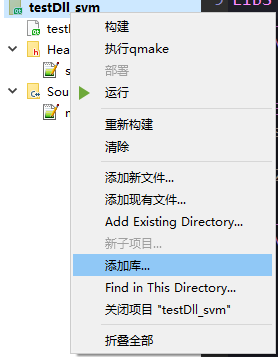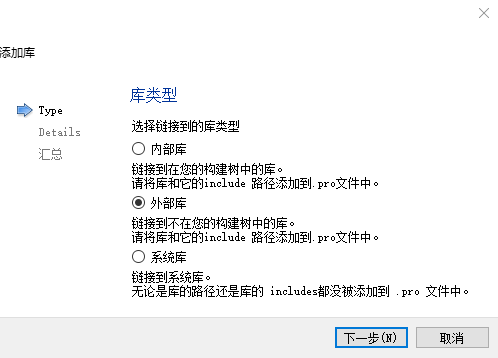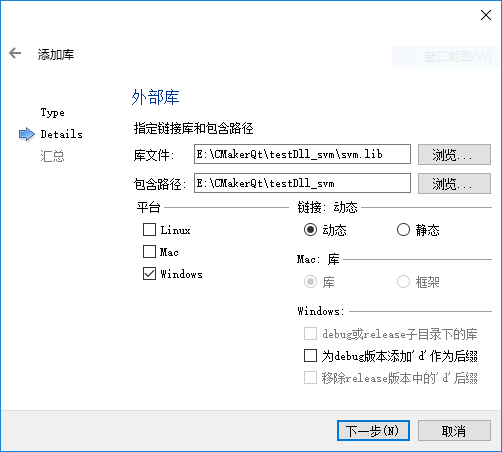点击下一步，你就会发现你的.pro文件中多了几行： win32: LIBS += -L$$PWD/./ -lsvm

INCLUDEPATH += $$PWD/. DEPENDPATH +=$$PWD/.
自己直接写也可以，但前提是你得写对哦~
3、添加matlab依赖以及头文件
这个就相对简单粗暴了，首先确认你的matlab路径，然后复制粘贴即可
LIBS += -LD:/matlab2015b32/extern/lib/win32/microsoft -lmclmcrrt
LIBS += -LD:/matlab2015b32/extern/lib/win32/microsoft -lmclmcr
LIBS += -LD:/matlab2015b32/extern/lib/win32/microsoft -llibmx
LIBS += -LD:/matlab2015b32/extern/lib/win32/microsoft -llibmat
INCLUDEPATH += D:/matlab2015b32/extern/include
DEPENDPATH += D:/matlab2015b32/extern/include
我的……就这么多就够了，网上有不少恨不得把所有的库都填进去的……也没差，反正这年头你也不会一个字母一个字母地往上打，但这里就涉及到一个问题，应该放多少呢……不知道，我真地不知道，反正我的就这么几行，程序好使，没报错，嗯。
当然还要注意，在你的头文件中刚才你拷进去的.h文件
4.动态调用
QLibrary myLib("svm.dll");
typedef bool MW_CALL_CONV(*Fun)(int,class mwArray &,class mwArray const &,class mwArray const &,class mwArray const &);
Fun svm = (Fun)myLib.resolve("?svm@@YAXHAAVmwArray@@ABV1@11@Z");
这里需要注意，加粗上红字，嗯，emmmmmmmmmm
分条说：
1、我之前说过dll文件和代码放一起吧，就是这样，不放一起找不到
2、你需要一个软件 dependency walker，这个软件是干嘛用的呢……看dll文件中的函数用的，别慌，你别慌，别关网页，这个真不难，咱都是用matlab的，都不是计算机科班出身，我都学会了你还能学不会？
这玩意就几M，界面是这样的你唯一需要的就是左上角的打开按钮，使用它打开dll文件就这么简单，打开你生成dll文件你会发现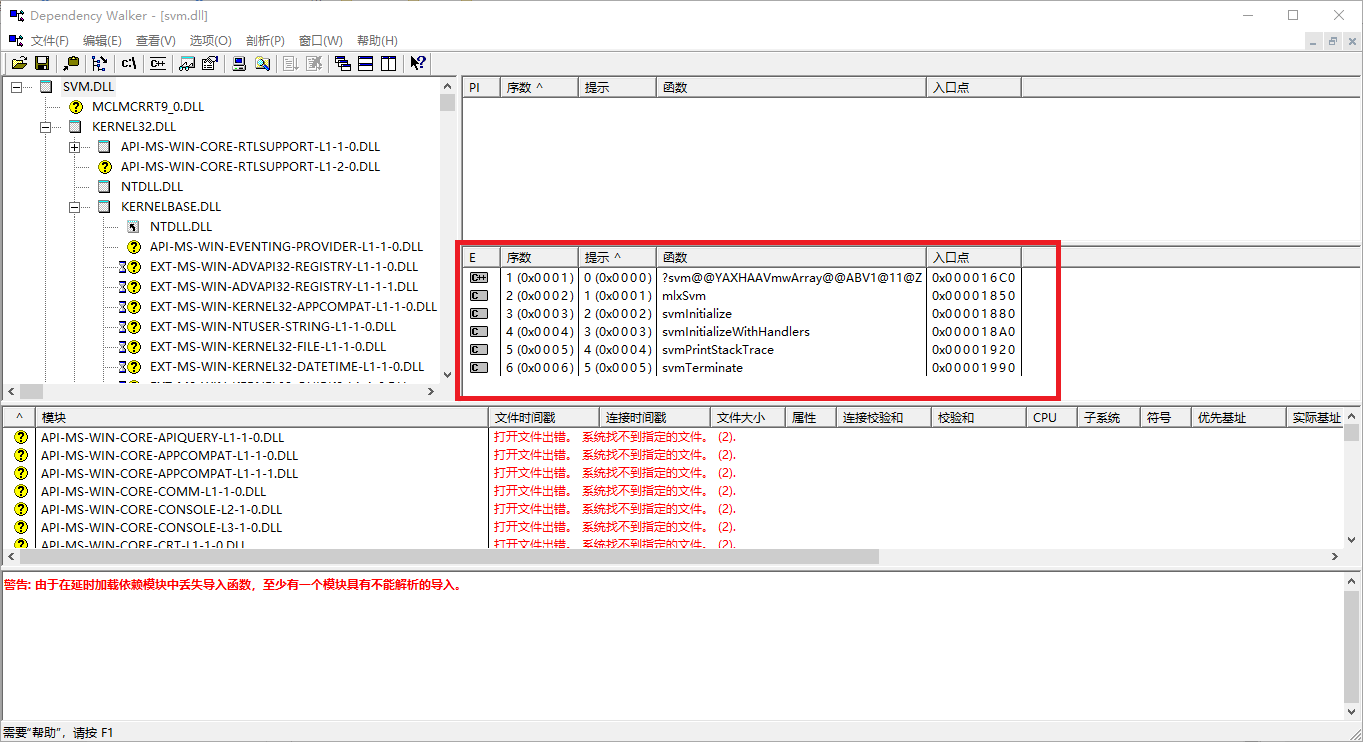你会发现乱码中夹着你写的函数，我的是svm，什么你的不是乱码？那你右键点一下选择取消修饰C++函数看看，必乱码。
这是由于C++的重载，用形参列表来区分同名函数，因此在调用时对函数名进行了编码（自己的理解，有大老知道请指教）。
这是由于matlab使用vs的msvc编译器生成的dll文件，生成后我们使用mingW调用，但生成的头文件中指定各种编译器对应的情况，但唯独没有MinGW，这就导致了在使用MinGW编译器时我们使用了：
DEFINES += __MW_STDINT_H__
使用msvc编译器调用matlab生成的dll时，编译器会自动识别函数名，因此也不需要使用resolve函数，这一点博主会在后续博文中详细介绍。
但现在既然乱码了我们怎么处理呢，你看我上面那几行就完事，其实就是用Qlibrary的resolve函数把乱码换个函数名，仅此而已，乱码可以从dependency walker中直接复制过来，函数原型当然也要对应着你自己的修改一下。
剩下的，完事了，再不完事这个代价就有点大了，讲道理我费这么大力气（其实也没……还好）做出dll，实际执行速度什么的我还没测试，这玩意……确实有点麻烦。
emmmmmmmmmmm总结
感谢大佬qq657863206，是他将以上方法总结出来，由我总结发出来的。
那么回过头来，qt调用matlab动态链接库好处在哪？
1、qt做界面，matlab做算法，二者相得益彰
2、相对于将算法C++化，这样做当然节约时间和成本
缺点：
1、程序执行速度尚未测试，尽管转换为dll，我个人认为matlab甚至是官方并不重视这一需求，毕竟你不能对脚本语言期望太高，因此我对其执行效率表示担忧，不知道有没有人了解这一块，看在我码了这么多字的面子上能在评论区指点我一下。
//19.4.22更新/
最近看到网上有很多不需要使用Qlibrary和reslove给函数改名的程序，这是由于他们qt用的msvc编译器，再用msvc编译器调用就不会出现需要改名的问题。
如果你仔细看matlab的头文件你就会发现，其中考虑了不同平台不同编译器的情况，但是唯独没有使用mingw的情况，这是mathwork和ms这种闭源对开源的抵制？？？瞎猜的，别当真（手动滑稽）。

展开全文• 工具：MATLAB R2014b，Qt 5.6.1, 目标：通过MATLAB写一个简单的函数，生成动态链接库DLL，再在Qt上调用 1.在MATLAB主页新建一个函数 记住函数的名字保存的函数文件的名字要相同，比如我写了一个函数f，保存时...
工具：MATLAB R2014b，Qt 5.6.1,
目标：通过MATLAB写一个简单的函数，生成动态链接库DLL，再在Qt上调用
1.在MATLAB主页新建一个函数
记住函数的名字和保存的函数文件的名字要相同，比如我写了一个函数f，保存时文件名需要是f.m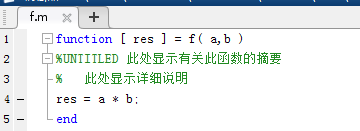2.生成动态链接库

首先要查看一下自己的MATLAB有没有编译器，在命令行输入!mcc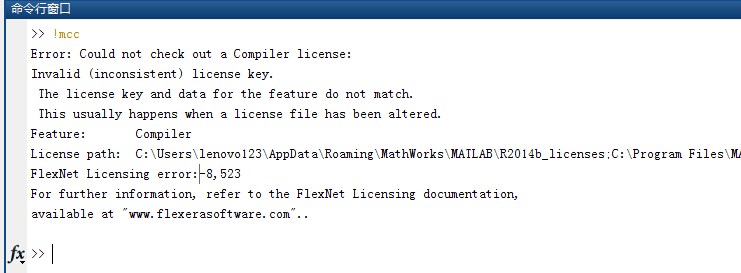好像是没有破解完全的原因，后来卸载重新破解了2014b，还是同样的问题，后来使用了mex命令出现了以下的错误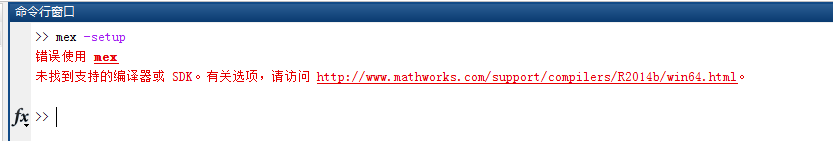好像是我压根就没有安装编译器，进入MATLAB给的网站，
http://www.mathworks.com/support/compilers/R2014b/win64.html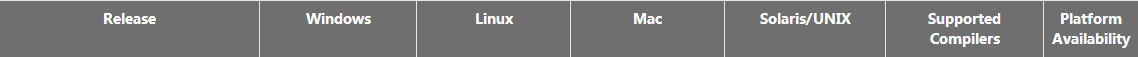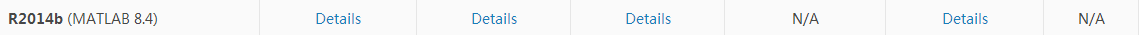选择R2014b的Supported Compilers下面的Details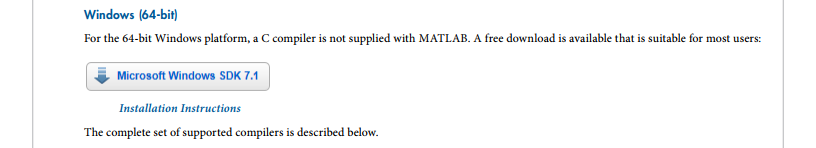安装完之后，又出现了以下问题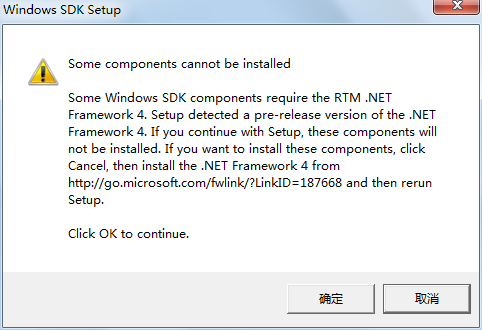安装了很久很久的.NET Framework 4.7.1 进度条没有丝毫要前进的迹象，上网查了一下，安装.NET Framework 4.7.1 似乎还要顺带安装Win 10 Creator Update 以及VS 2017，其中要勾选. NET  Framework 4.7 development tools...
后来擅自决定去下载. NET Framework 4.5（为什么事情变得越来越复杂了...）
安装的时候却提示已经下载好高版本的Framework，后来点击原来的SDK，却可以正常安装了，但是进度条也是，纹丝不动。
后来结束了上面的进程。后来下载了SDK镜像，还是安装不了。
后来去控制面板处发现以前装VS2015的时候已经下载过高版本的SDK了。并不需要再安装SDK = =
可能是网上都用20个1这个激活码，都没有下载编译器。所以决定卸载MATLAB，用其他的激活码重新激活。
用其他激活码激活后，出现了一下问题。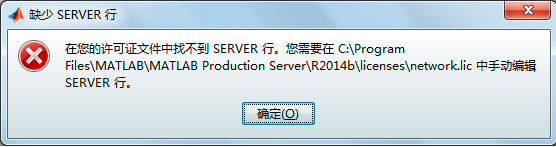但是，再次进入MATLAB的时候又出现了新的问题。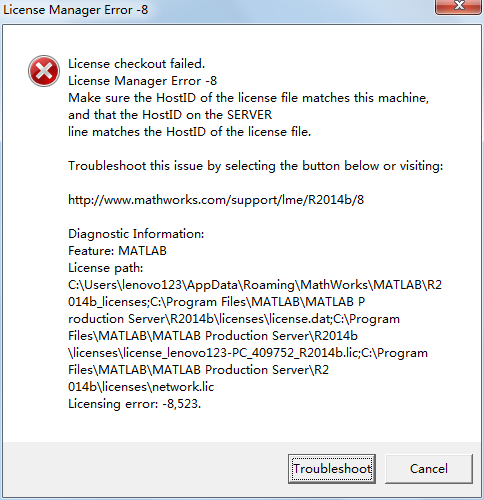问题解决：在网上的crack文件中将“libmwservices.dll”文件替换“...\matlab\bin\win64”下的“libmwservices.dll”
然后可以顺利进入MATLAB，但是仍然没有编译器。在网上看到了无数人都出现了和我一样的情况，但是好像都没几个人真正解决。
解决方法：换一个被完全破解的MATLAB，这里我选择了MATLAB R2014a，百度云资源真是非常难找了。
安装激活matlab2014a后，出现了新的问题。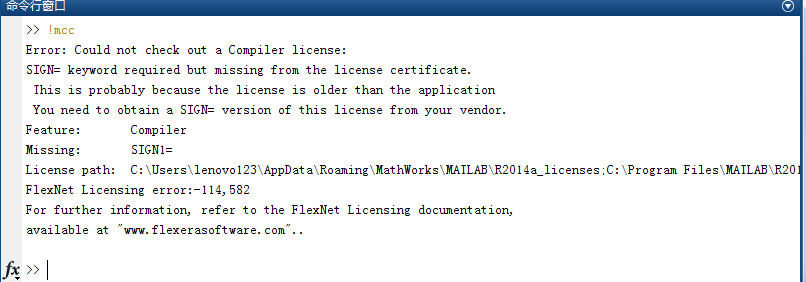后来发现原来是我有几个文件没有替换，换掉之后就好了。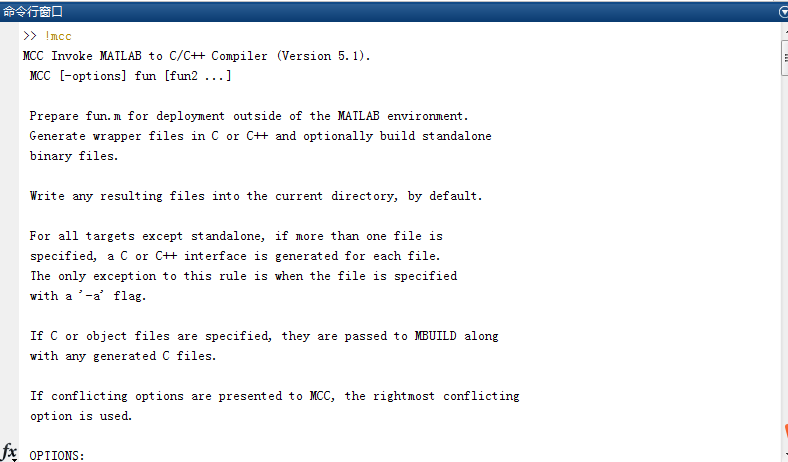旧的不去新的不来，又出现了新的问题。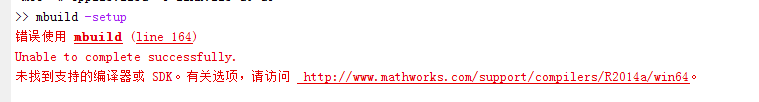这篇博客有介绍MATLAB 2014a VS2015找不到编译器的问题https://blog.csdn.net/hzd12368/article/details/70526955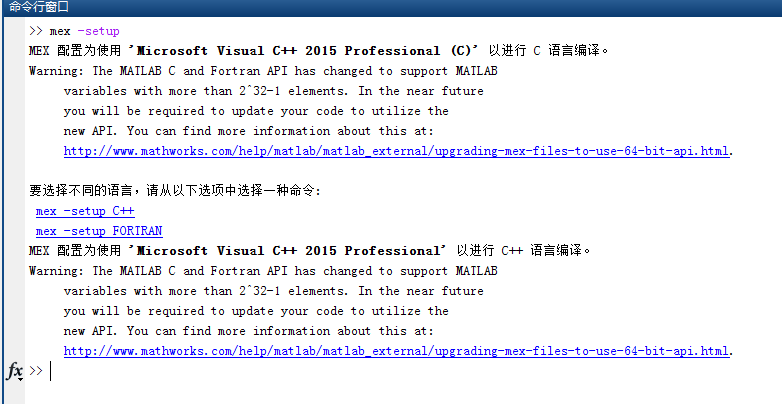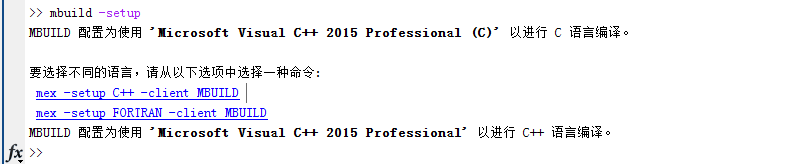都选择C++那个选项
在存放函数的目录下，在命令行输入mcc -W cpplib:libf -T link:lib f.m -C，（这是生成f.cpp的命令）

mcc -W lib:libf -T link:lib f.m -C（这个应该是生成f.c的命令）时间可能较长，大约一分钟左右，生成了如下图的文件。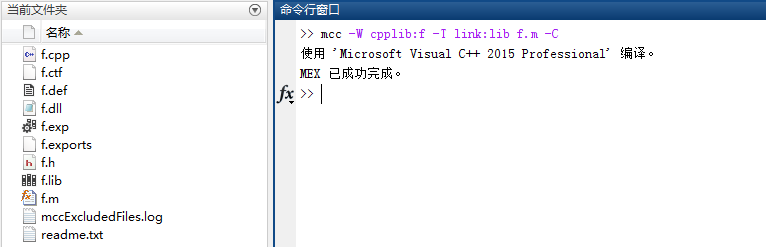至此，动态链接库就已经生成了。
3.QT调用MATLAB函数
将.h和.lib文件拷到QT的工程目录下。将QT工程运行一下会生成debug文件，将.dll和.ctf文件复制到debug文件下。
将MATLAB lib路径、头文件等写入Qt .pro文件：
注：若路径中有空格写pro文件的格式会略有变动
此处参考博客https://blog.csdn.net/qq_33221028/article/details/78398408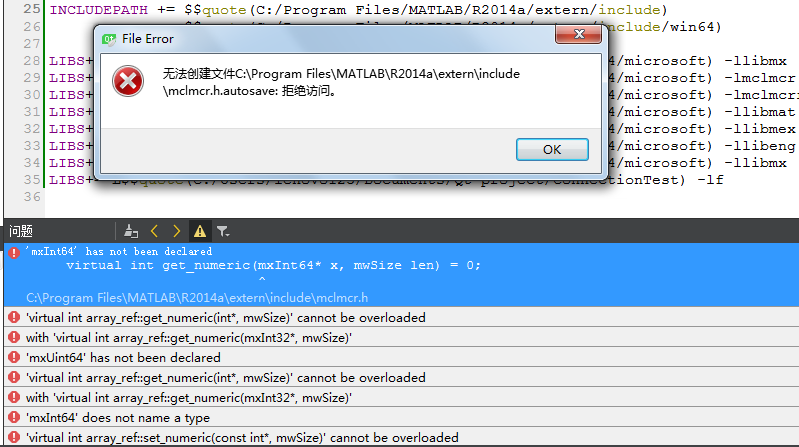后来在某个大佬的博客上发现了解决办法：即在.pro文件中添加
DEFINES += __MW_STDINT_H__总体的pro
#-------------------------------------------------
#
# Project created by QtCreator 2018-04-10T09:59:54
#
#-------------------------------------------------

QT       += core gui

greaterThan(QT_MAJOR_VERSION, 4): QT += widgets

TARGET = CTest2
TEMPLATE = app

SOURCES += main.cpp\
mainwindow.cpp

f.h

FORMS    += mainwindow.ui

OTHER_FILES += f.ctf

INCLUDEPATH += $$quote(C:/Program Files/MATLAB/R2014a/extern/include)$$quote(C:/Program Files/MATLAB/R2014a/extern/include/win64)

LIBS+=-L$$quote(C:/Program Files/MATLAB/R2014a/extern/lib/win64/microsoft) -llibmx LIBS+=-L$$quote(C:/Program Files/MATLAB/R2014a/extern/lib/win64/microsoft) -lmclmcr
LIBS+=-L$$quote(C:/Program Files/MATLAB/R2014a/extern/lib/win64/microsoft) -lmclmcrrt LIBS+=-L$$quote(C:/Program Files/MATLAB/R2014a/extern/lib/win64/microsoft) -llibmat
LIBS+=-L$$quote(C:/Program Files/MATLAB/R2014a/extern/lib/win64/microsoft) -llibmex LIBS+=-L$$quote(C:/Program Files/MATLAB/R2014a/extern/lib/win64/microsoft) -llibeng
LIBS+=-L$$quote(C:/Program Files/MATLAB/R2014a/extern/lib/win64/microsoft) -llibmx LIBS += C:\Users\lenovo123\Documents\MATLAB\test2\c++\f.lib DEFINES += __MW_STDINT_H__ unix:!macx|win32: LIBS += -L$$PWD/./ -lf

INCLUDEPATH += $$PWD/. DEPENDPATH +=$$PWD/.
pro最后三行是我导入了外部库所生成的代码，就是将f.lib导入到工程
用c和cpp文件都尝试过，最后都无法避免的出现了undefined reference to ‘fInitialize’等error
void MainWindow::on_pushButton_clicked()
{
if(!fInitialize())
QMessageBox::warning(this,tr("error"),tr("fail to initialize lib"),QMessageBox::Ok);
QString result;
double para1,para2;
para1 = ui->inputEdit1->text().toDouble();//获取输入框的第一个数
para2 = ui->inputEdit2->text().toDouble();//获取输入框的第二个数
mwArray a(1,1, mxDOUBLE_CLASS);
mwArray b(1,1, mxDOUBLE_CLASS);
mwArray c(1,1, mxDOUBLE_CLASS);
a(1,1) = para1;
b(1,1) = para2;
f(1,c,a,b);
/*
double _x,_y;
_x = para1;
_x = para2;
mxArray *input = mxCreateDoubleMatrix(1,2,mxREAL);
mxArray *output = mxCreateDoubleMatrix(1,1,mxREAL);
memcpy(mxGetPr(input),(void *)_x,sizeof(_x));
mlxF(1,&output,1,&input);
memcpy(_y,(double *)mxGetPr(output),sizeof(_y));
*/
/**
* 注：1.qt的lineEdit编辑框上接收的是QString类型，所以输入输出都要转换成需要的数据类型
*     2.C++向MATLAB传参数的时候是以矩阵的形式传递，单个数值算1*1的矩阵
*     3.fInitialize(),f(),mlxF(),fTerminate()这些函数都是f.c/f.cpp中写好的函数，f.h中都有定义
*/
/**ui->resultEdit->setText(result);//在编辑框中输出结果**/
fTerminate();
}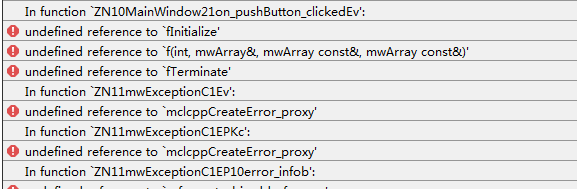以上错误仍没有解决，如有大佬知道怎么做，麻烦不吝指教，感激不尽。
把所有代码都附上，希望有人能够解决我的问题，留言告诉我，3q
主要参考的博客还有：https://blog.csdn.net/Althindor/article/details/77679303
历时3天，最后放弃使用Qt了，转向使用Matlab写界面。
展开全文• ## Qt与Matlab混合编程细节总结

万次阅读 多人点赞 2017-10-30 22:51:23
相关的文章CSDN上并不少，但是自己实践为什么总不能成功呢？答曰：Qt的pro文件细节太多，要全面把握方可成功实现混合编程。第一次掌控细节后，以后用起来就顺手多了。mwArray
• Matlab函数转Qt操作步骤Matlab函数转Qt具体操作步骤1.打开脚本文件选择编译器编译选项生成需要的库文件在Qt中的使用创建一个表格设定内容居中、居左、居右SmartyPants创建一个自定义列表如何创建一个注脚注释也是必...
• Matlab函数转Qt具体操作步骤，链接： QtMatlab库函数的使用 在Qt中使用Matlab编译的文件 设置Qt工程中的.pro文件 将Matlab生成的lib,h,dll拷贝到当下工程路径内。 在.pro文件的空白处单击右键，弹出库...
• QtMATLAB混合编程 前言：本文主要讲述以Qt来开发，MATLAB做数学计算，c++来编写程序，将计算的结果生成相应的库文件或者直接生成一个可执行文件给Qt调用，以此来实现QtMATLAB混合编程。 环境概述： 操作...c++
• QtMatlab 混合编程在网上可以找到资料，自己找了很多资料后才把环境搭建好，还是总结一下方便以后使用。 Matlab 编译 C++ 库 Qt 调用 Matlab 库 注意事项 环境搭建 Windows 10 企业版 Matlab2019b-64bit Qt ...
• 最近要开发个信号处理软件，信号处理的算法是Matlab程序编写的。现在涉及到Qt 和Matlab 混合编程的问题，希望有做成功的大神可以指点指点。算法
• 总结网上有些大神做的Qt混编matlab的过程，中间有些步骤出现不一样或者有问题的地方，自己总结并实例实现一个拟合曲线并显示的过程。
• ## QTcreator&Matlab混合编程

千次阅读 热门讨论 2018-10-23 21:30:09
因为项目的需要这两日一直在摸索matlabqtcreator混合编程的东西，期间遇到过很多的问题，在此进行一个总结，希望能够帮到后面有需要的人。 环境：Windows10 64位、Qtcreator5.7 32位、Matlab2014b 32位（注意：...
• Qt5.15.0和Matlab2020b混合编程1.软件环境1.1 Qt编译器1.2 Matlab2020b1.3 Visual Studio 20192..m文件编译成c++的DLL文件2.1Matlab中简单的m文件编写2.2mbuild -setup命令2.3mbuild –setup C++命令2.4deploytool...c++
• Qt Creator与matlab混合编程 引言：项目要求在Qt平台下搭建基于有限元算法的光学仿真平台，首先需要进行网格划分的实现。由于网格划分算法在matlab平台下已经有非常成熟的工程以及代码，如果可以直接调用，将大大...
• 一、概要 ...简单来说，可以QtMatlab共同读写同一文件，比如Qt将原始数据放入文件，Matlab检测到后对原始数据进行计算，然后将结果放到这个文件中供Qt读取。 2. Matlab的m文件可以编译为Qt可以调用的c++...

# qt和matlab混合编程matlab 订阅